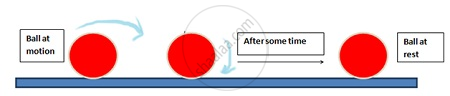Share

# Aristotle’s Fallacy

## Aristotle’s fallacy:

Aristotle, the Greek Scientist held the view that an external force is required to keep a body in uniform motion.
His concept is outdated now, because he considered only one side of motion and fails to explain the other i.e. if body is in motion then how does it come to rest? There came the concept of the opposing external force of Friction.

What is the flaw in Aristotle’s argument?

• The answer is: a moving toy car comes to rest because the external force of friction on the car by the floor opposes its motion.

• To counter this force, the child has to apply an external force on the car in the direction of motion.

• When the car is in uniform motion, there is no net external force acting on it: the force by the child cancels the force (friction) by the floor.

• The corollary is: if there were no friction, the child would not be required to apply any force to keep the toy car in uniform motion.

• The opposing forces such as friction (solids) and viscous forces (for fluids) are always present in the natural world.

• This explains why forces by external agencies are necessary to overcome the frictional forces to keep bodies in uniform motion.

• Now we understand where Aristotle went wrong. He coded this practical experience in the form of a basic argument. To get at the true law of nature for forces and motion, one has to imagine a world in which uniform motion is possible with no frictional forces opposing. This is what Galileo did.

For example, a ball rolled on the floor comes to rest after some time due to opposing force of frictionConclusion:
An external force is required to keep a body in motion, only if resistive forces (like frictional & viscous forces) are present.

S